# Quantum Phase Mechanics: A Hypothesis

Some of my beliefs regarding the theory which underlies both Quantum Mechanics and Special Relativity...SiteMap of SciRealm | About John | John's Resume/C.V./Bio | Send email to John John's Science, Math, Philosophy Stuff: The Science Realm: John's Virtual Sci-Tech Universe | 4-Vectors | Ambigrams | Antipodes | Covert Ops Fragments | Cyrillic Projector | Forced Induction (Sums Of Powers Of Integers) | Fractal Tree | Frontiers | IFS Fractals (Assembly) | IFS Fractals (Javascript) | JavaScript Graphics | Kid Science | Kryptos | Philosophy | Photography | Physics-Planck Constant Measurement Via LEDs | Prime Sieve | QM from SR | QM from SR-Simple RoadMap | QM from SR-Simple RoadMap (PDF) | SR 4-Vector & Tensor Calculator |Quantum Phase | Quotes | RuneQuest Cipher Challenge | Secret Codes & Ciphers | Scientific Calculator (complex capable) | Science+Math | Sci-Pan Pantheist Poems | Stereograms | SuperMagicSqr4x4 |Turkish Grammar |Melike Wilson Art Site last modified: 2021-Aug Welcome to Relativistic Quantum Reality: Virtual worlds of imaginary particles: The dreams stuff is made of: Life, the eternal ghost in the machine... This site is dedicated to the quest for knowledge and wisdom, through science, mathematics, philosophy, invention, and technology.  May the treasures buried herein spark the fires of imagination like the twinkling jewels of celestial light crowning the midnight sky... Quantum Mechanics is derivable from Special Relativity See SRQM - QM from SR - Simple RoadMap (.html) See SRQM - QM from SR - Simple RoadMap (.pdf) See SRQM - Online SR 4-Vector & Tensor Calculator See Online Complex-capable RPN Scientific Calculator ***Learn, Discover, Explore***Note: This is a work in progress...if you notice errors, please let me know.

Abbreviations
QM=Quantum Mechanics
SR=Special Relativity
GR=General Relativity

Introduction

There are still several fundamental problems with the interpretation of Quantum Mechanics. Admittedly, it is arguably the most successful theory in all of physics, but there are a number of paradoxical and unintuitive results that occur when the formalism is blindly followed. It is my opinion that QM as currently formulated is not a final theory. I believe that there is a deeper structure which gives QM as a statistical approximation, just as Classical Mechanics is an approximation of QM for systems composed of many particles.

Let's begin with the measurement problem.

A "observed" system makes a discontinuous "jump" into a single eigenstate of the measurement observable. Which of the final states will be selected is currently an unknown and unknowable in standard QM.

However, this system + observer is just a subset of a larger "unobserved" system, which evolves deterministically according to the Schrodinger equation, or more accurately the Klein-Gordon or Dirac equations, its relativistic cousins. Based on this, the device for making a measurement "selection" should reside within the QM formalism, without having to ad hoc in a new "hidden variable".

Everything is made of quantum particles. I do not subscribe to Bohr's artificially drawn line between quantum and classical systems.

I propose that the overall phases of single quantum particles are the devices by which measurement selections are made.

What are the properties which support this?

1) QM has been enormously successful over the past years for predicting all kinds of phenomena. Any successor theory must give the same results within the valid regime of QM. The overall phase does not change the probabilistic predictions of QM.

Let |S> = eia |s>, then <S|S> = <s|e-ia eia |s> = <s| e-ia+ia |s> = <s| e0 |s> = <s|s>

Hence, as stated in so many QM books, the overall phase plays no role in the probabilistic predictions of QM. However, this does not rule out the possibility that the overall phase could play some role in which eigenstate is selected in a measurement process. It simply puts a condition on the allowed values that the phase might take - it must obey a statistical law if one repeats "identical up to a phase factor" experiments.

2) The overall phase is a relativistic invariant. Thus, all inertial observers will agree on phase values.

Phi = KR = wt - kr

Also, this relation gives the phase values as the particle moves through spacetime. Other relativistic invariants include such things as c-speed of light, m0-rest mass, hbar-Planck's constant, q-electric charge, etc.  Note that in the particle's own rest-frame, the overall phase will change according to wt. Thus the phase is always "spinning" around the time axis. If a particle has a constant K, one can show that:

Phi(K=K0) = (m0c2 / hbar) T + K0R0

This description shows how the phases of separate particles could maintain a phase entanglement. If the particles are of equal rest mass and if they begin at the same spacetime event point, then their respective phases change at the same rates according to each one's proper time.
3) When one considers free particle scattering theory, the result is that the only change in the wave function at large distances is a change in the phase of the outgoing wave. Think about this. We are repeating "identical up to a phase factor" experiments with two particles. We have a target particle at a certain location. We shoot a moving particle at it with the same velocity from the same location in each setup. QM can not predict the exact outcome of this simple experiment, just the scattering cross-section probabilities. What can determine the actualized outcome? There is apparently some sort of phase interaction that occurs in the collision. Perhaps the differing overall initial phases of the particles involved in the same experimental configuration are what account for the different possible outcomes of the same experiment.

References (on 4-Vectors in SR & QM in SR)
Classical Dynamics of Particles & Systems, 3rd Ed., Jerry B. Marion & Stephen T. Thornton (Chap14)
Classical Electrodynamics, 2nd Ed., J.D. Jackson (Chap11,12)
Classical Mechanics, 2nd Ed., Herbert Goldstein (Chap7,12)
A First Course in General Relativity, Bernard F. Schutz (Chap1-4)
Fundamental Formulas of Physics, Donald H. Menzel (Chap6)
Introduction to Electrodynamics, 2nd Ed., David J. Griffiths (Chap10)
Introduction to Special Relativity, 2nd Ed., Wolfgang Rindler (All) (**pg60-65,82-86**)
Lectures on Quantum Mechanics, Gordon Baym (Chap22,23)
Modern Elementary Particle Physics: The Fundamental Particles and Forces?, Gordon Kane (Chap2+)
Path Integrals and Quantum Processes, Mark Swanson (var)
Quantum Electrodynamics, Richard P. Feynman (Lec7-rest)
Quantum Mechanics, Albert Messiah (Chap20)
Quantum Mechanics and the Particles of Nature: An Outline for Mathematicians, Anthony Sudbery (Chap7)
Relativistic Quantum Fields, Mark Hindmarsh
The Theory of Spinors, E'lie Cartan (var)
Topics in Advanced Quantum Mechanics, Barry R. Holstein (Chap3,6,7) Email me, especially if you notice errors (which I will fix ASAP) or have interesting comments.## quantum relativity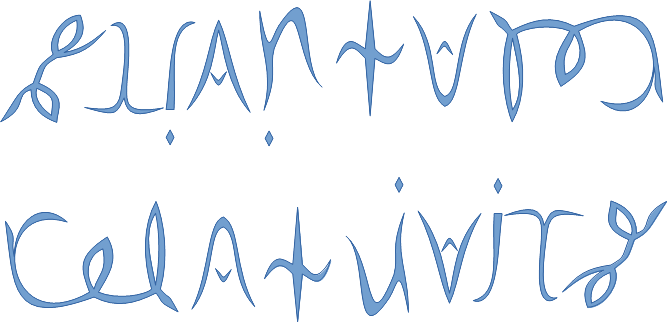See SRQM: QM from SR - The 4-Vector RoadMap (.html)
See SRQM: QM from SR - The 4-Vector RoadMap (.pdf)

SRQM Physics Diagramming Method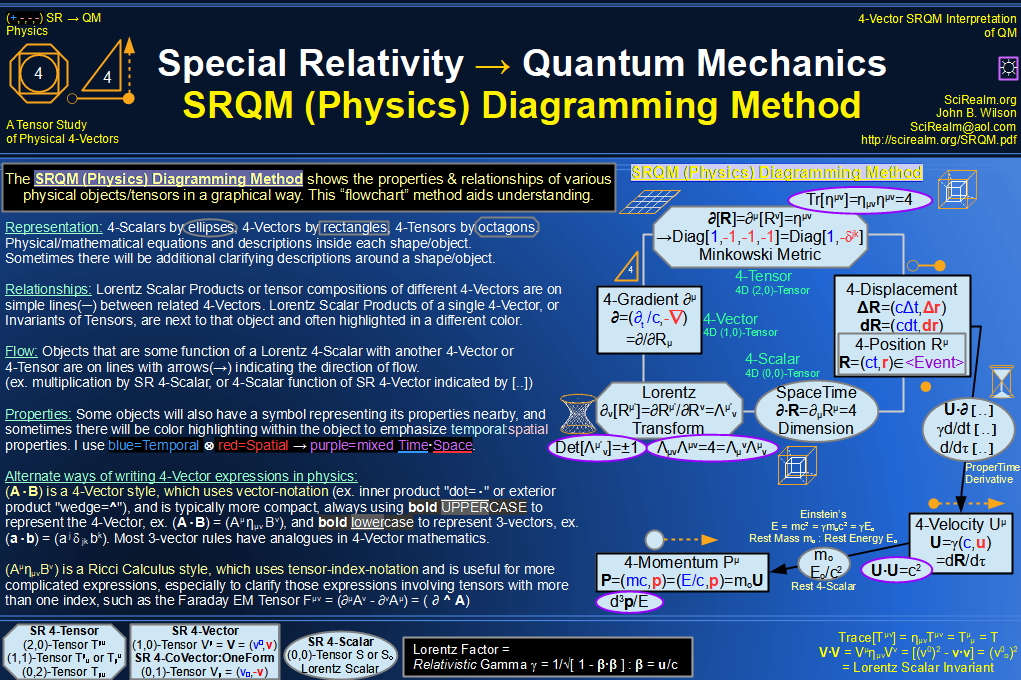SRQM 4-Vector : Four-Vector and Lorentz Scalar Diagram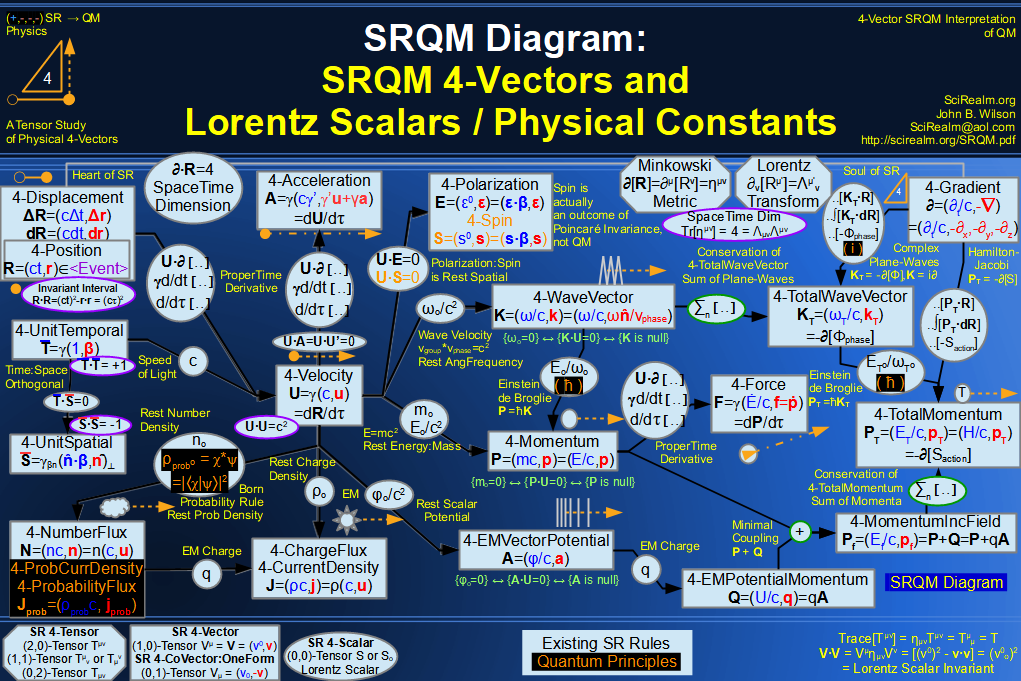SRQM + EM 4-Vector : Four-Vector and Lorentz Scalar Diagram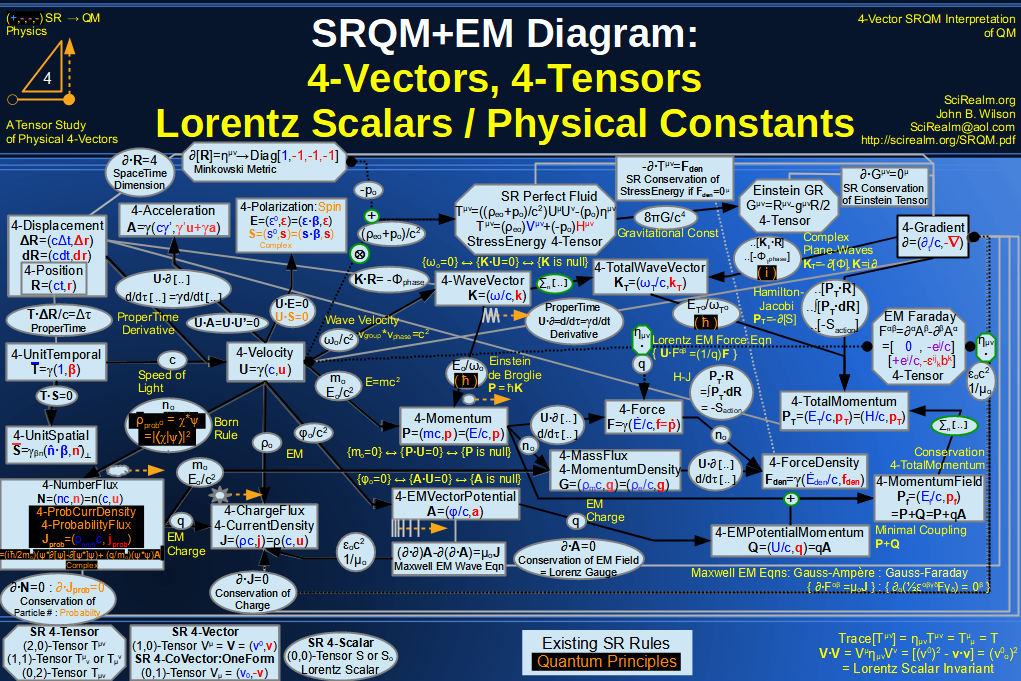SRQM + EM 4-Vector : Four-Vector and Lorentz Scalar Diagram With Tensor Invariants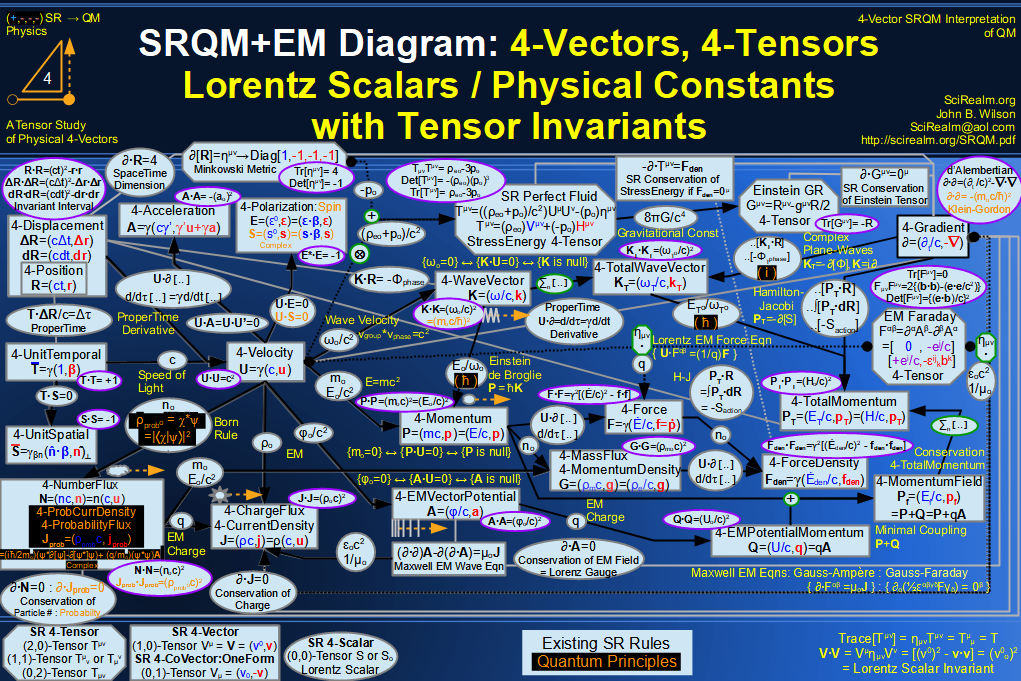SRQM 4-Vector : Four-Vector Stress-Energy & Projection Tensors Diagram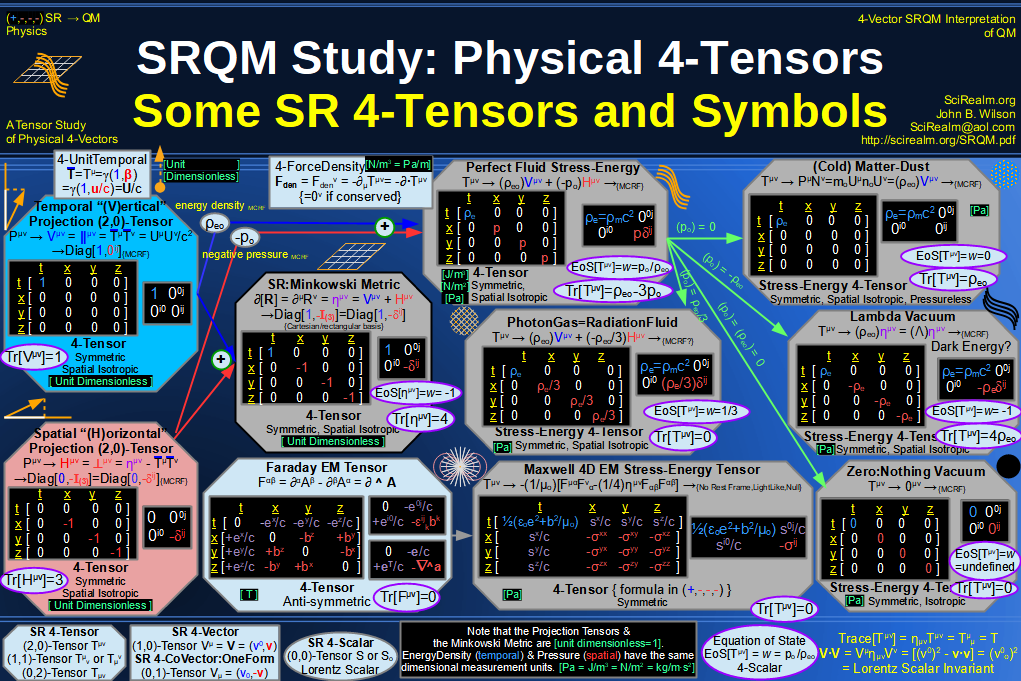SRQM 4-Vector : Four-Vector SR Quantum RoadMap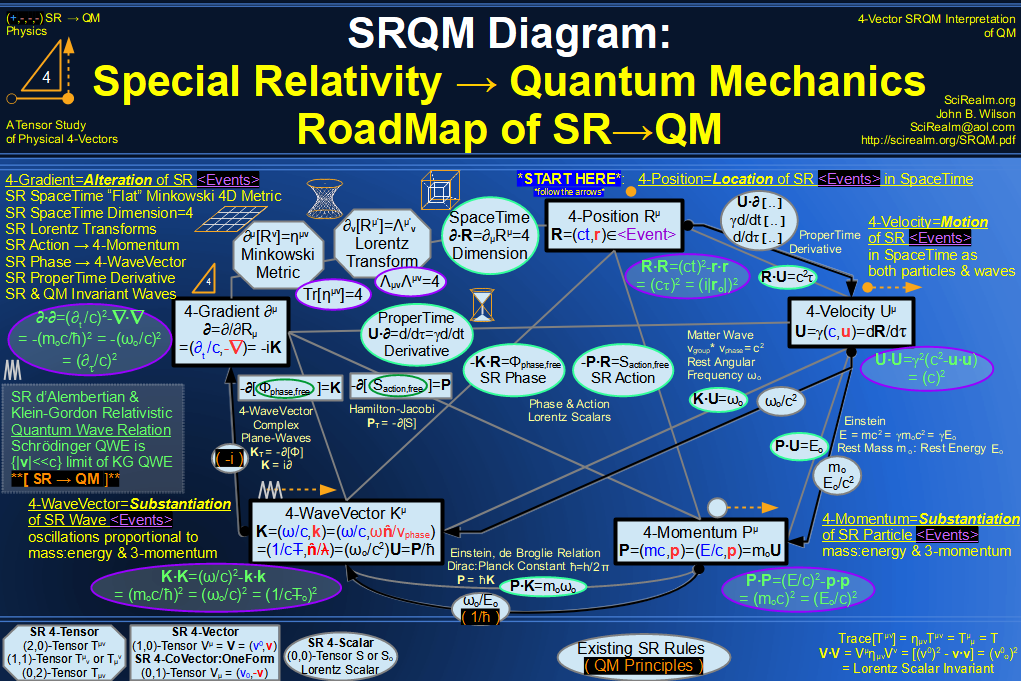SRQM + EM 4-Vector : Four-Vector SR Quantum RoadMap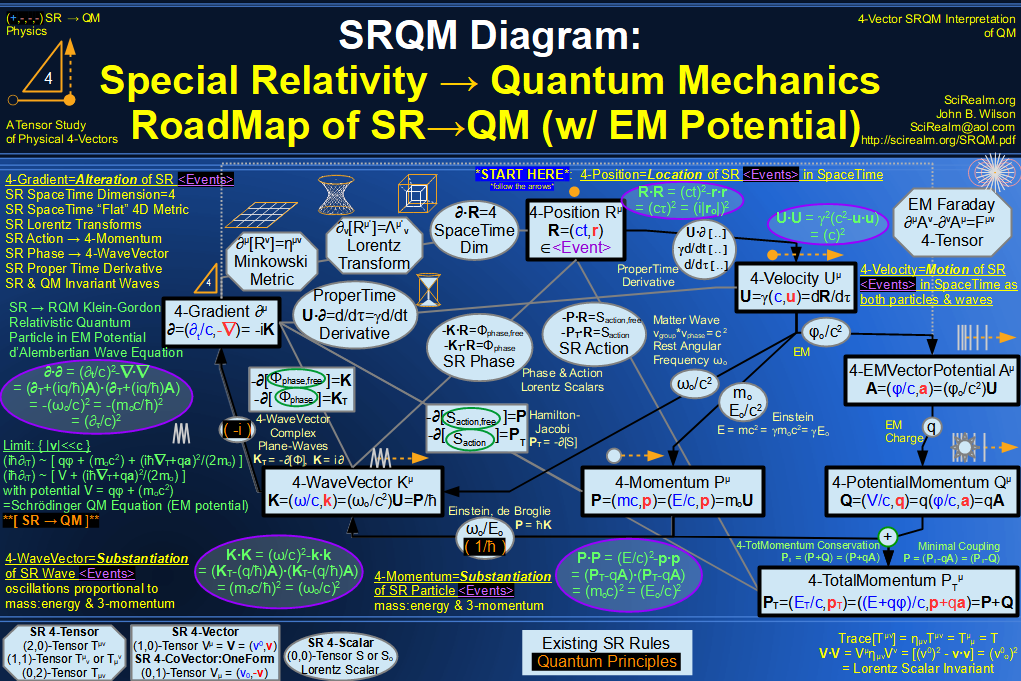SRQM + EM 4-Vector : Four-Vector SR Quantum RoadMap - Simple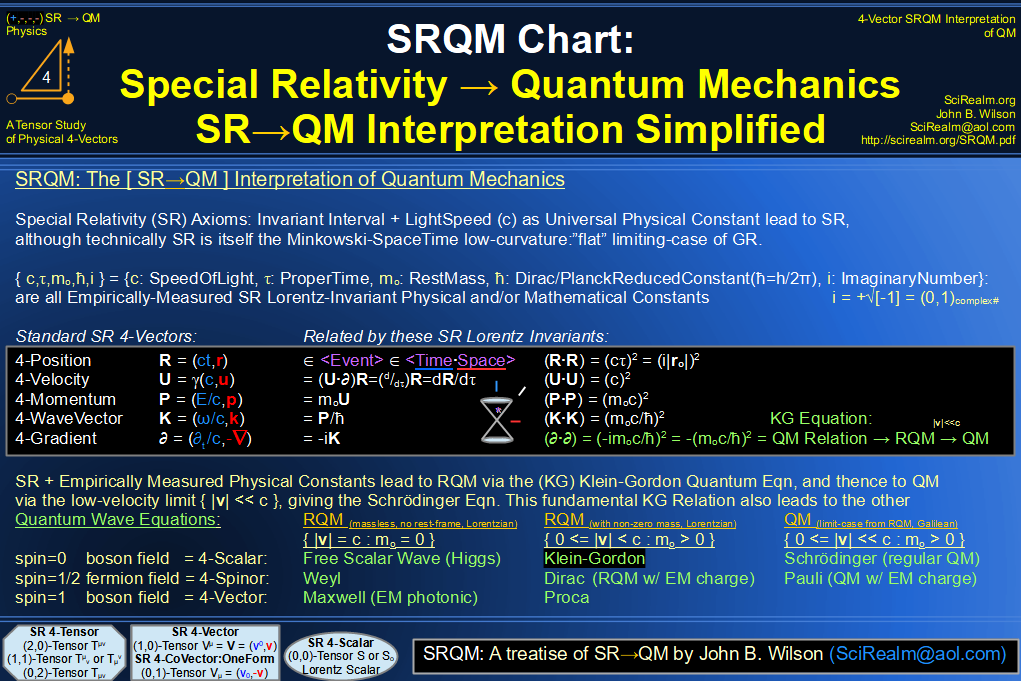SRQM 4-Vector : Four-Vector New Relativistic Quantum Paradigm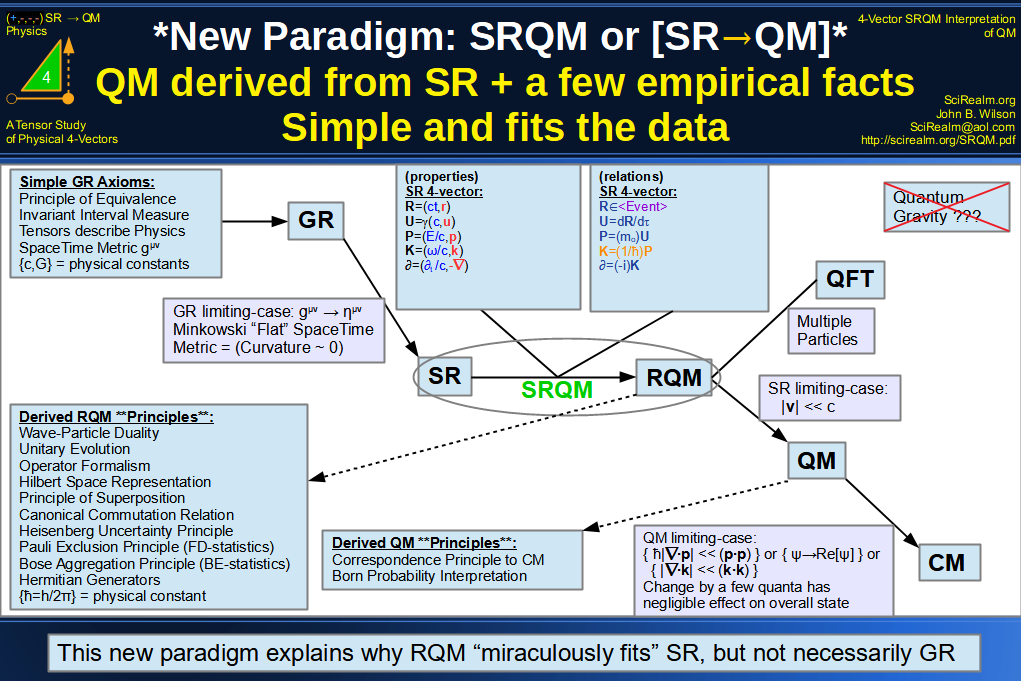SRQM + EM 4-Vector : Four-Vector New Relativistic Quantum Paradigm (with EM)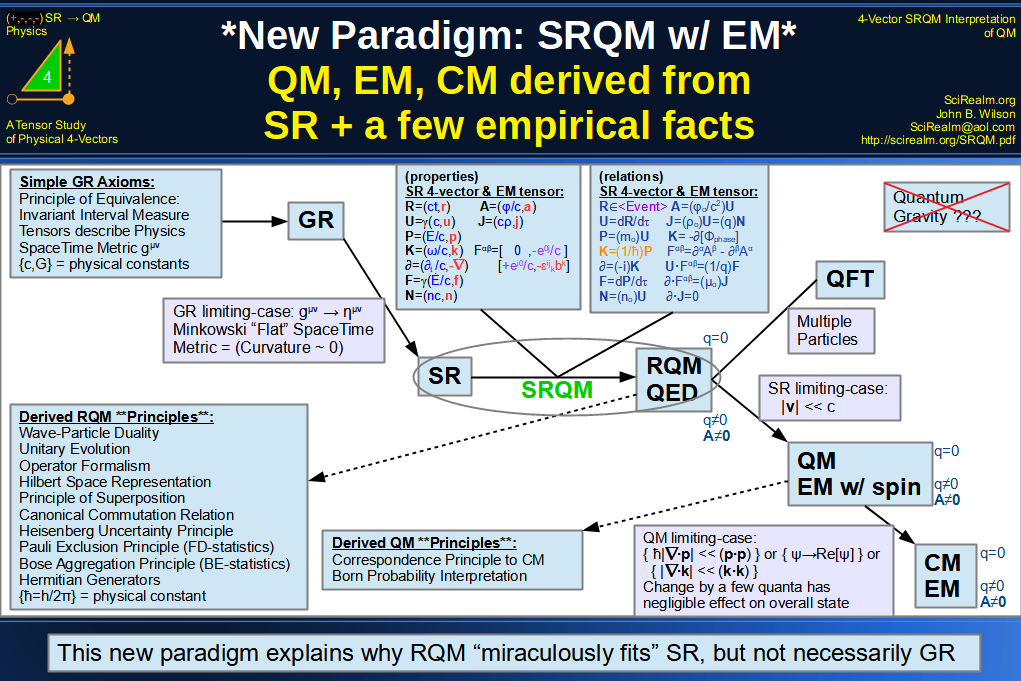SRQM 4-Vector : Four-Vector New Relativistic Quantum Paradigm - Venn Diagram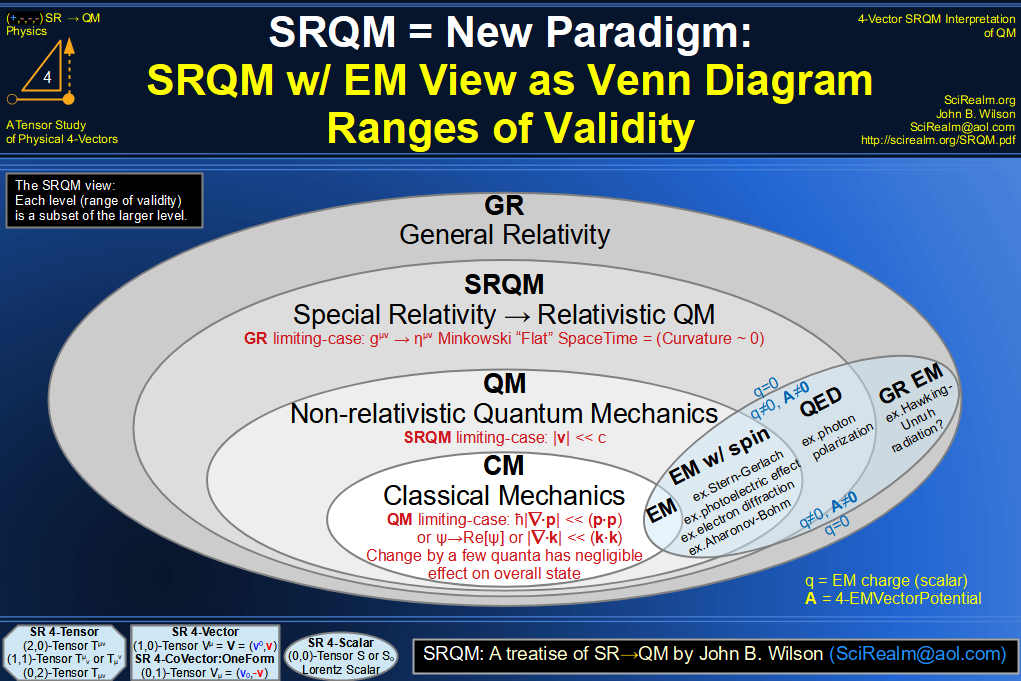SRQM 4-Vector : Four-Vector SpaceTime is 4D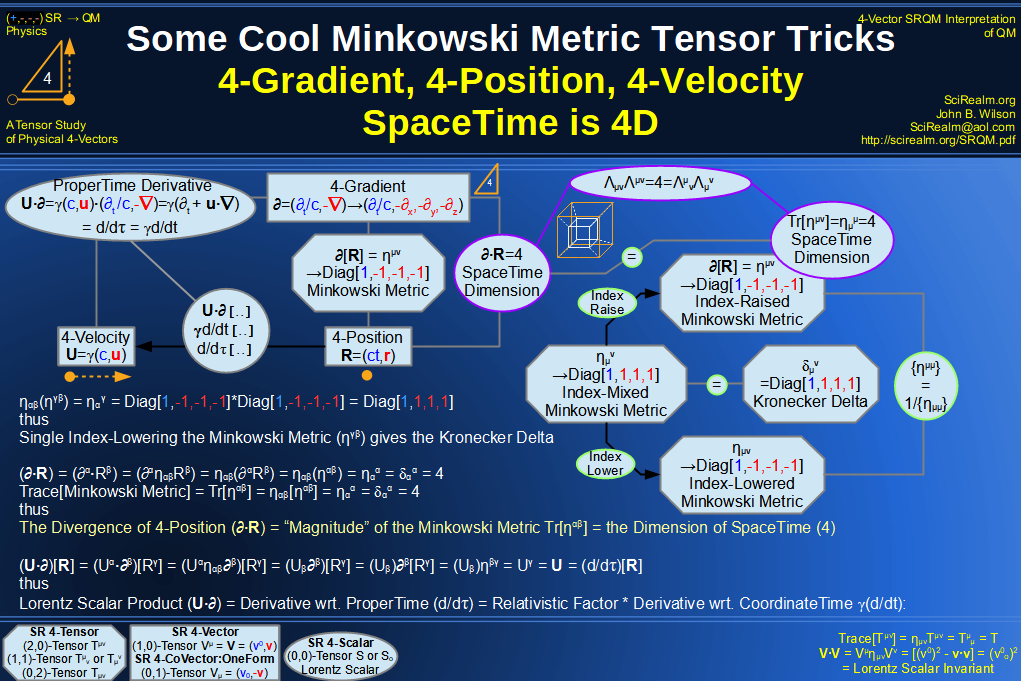SRQM 4-Vector : Four-Vector SpaceTime Orthogonality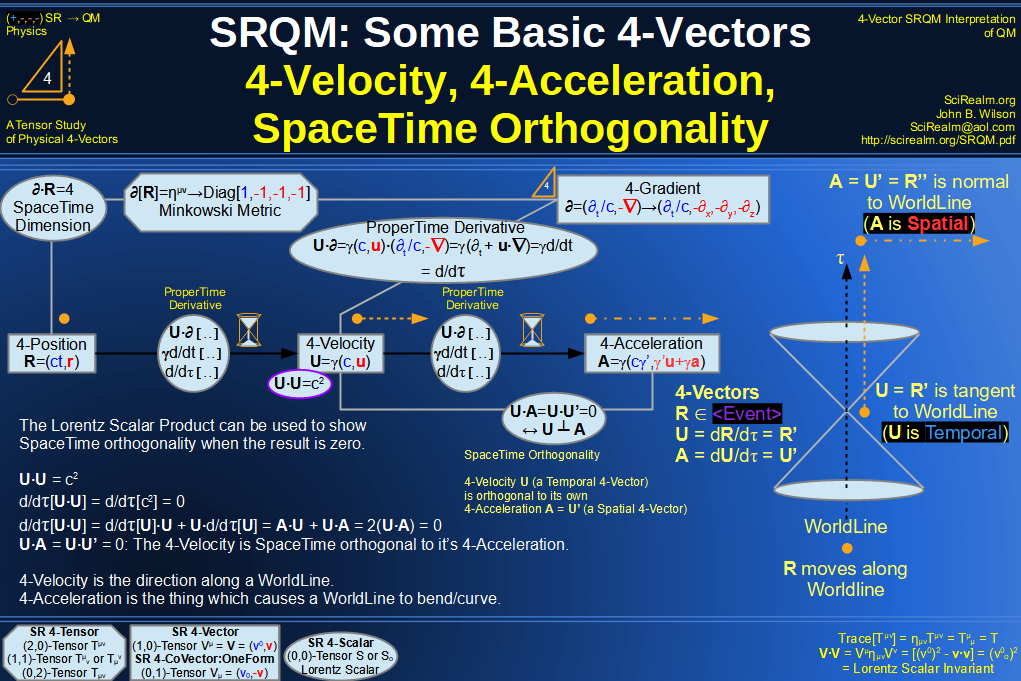SRQM 4-Vector : Four-Vector 4-Position, 4-Velocity, 4-Acceleration Diagram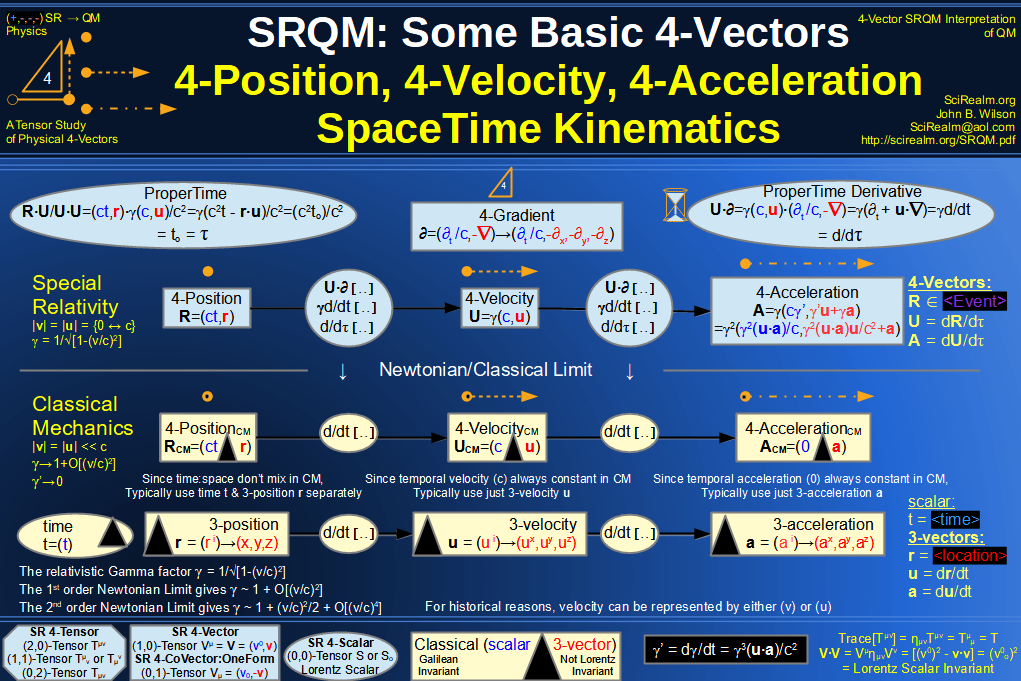SRQM 4-Vector : Four-Vector 4-Displacement, 4-Velocity, Relativity of Simultaneity Diagram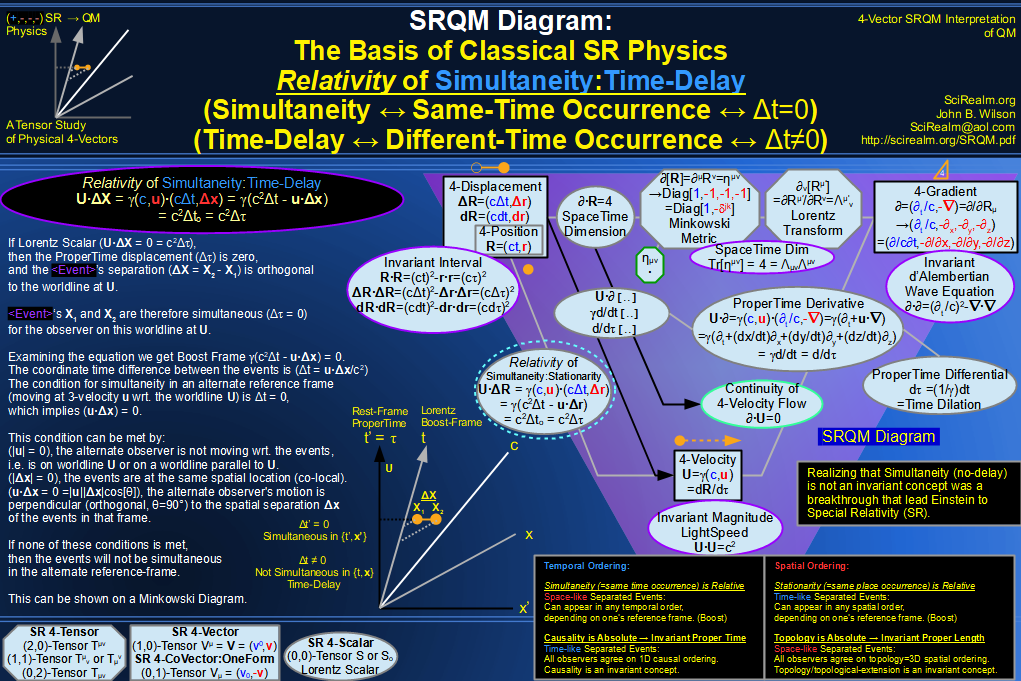SRQM 4-Vector : Four-Vector 4-Velocity, 4-Gradient, Time Dilation Diagram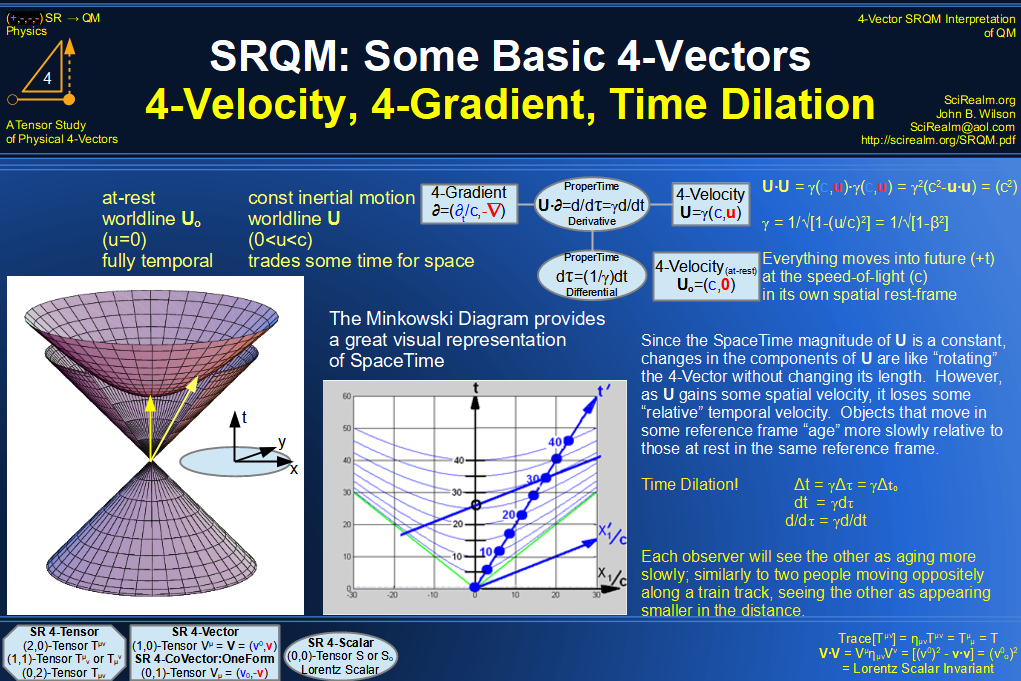SRQM 4-Vector : Four-Vector 4-Vector, 4-Velocity, 4-Momentum, E=mc^2 Diagram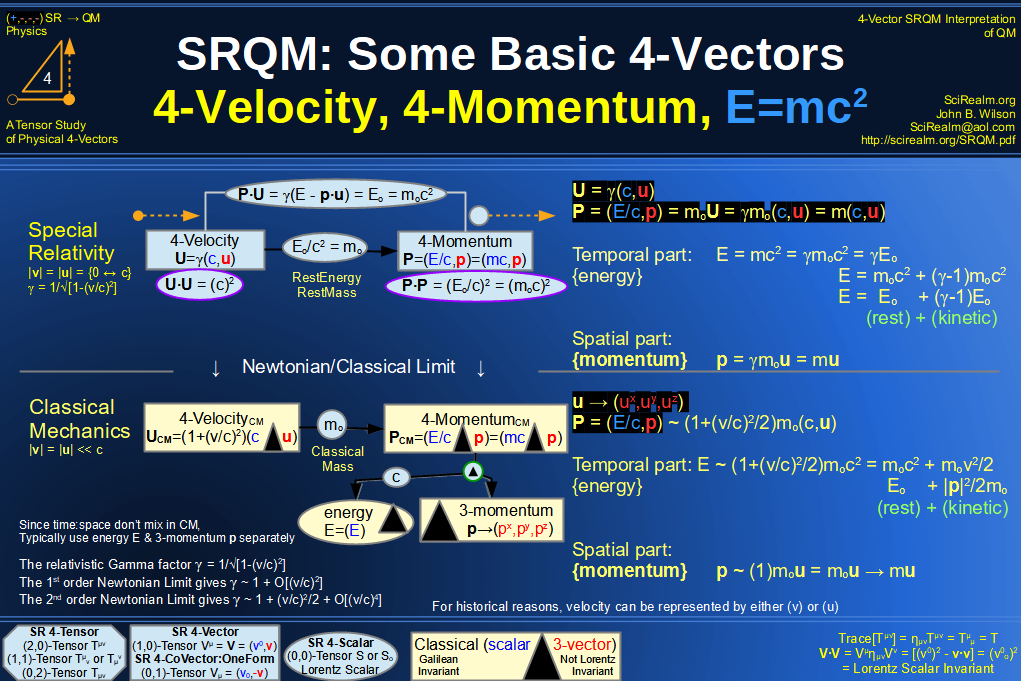SRQM 4-Vector : Four-Vector 4-Velocity, 4-WaveVector, Relativistic Doppler Effect Diagram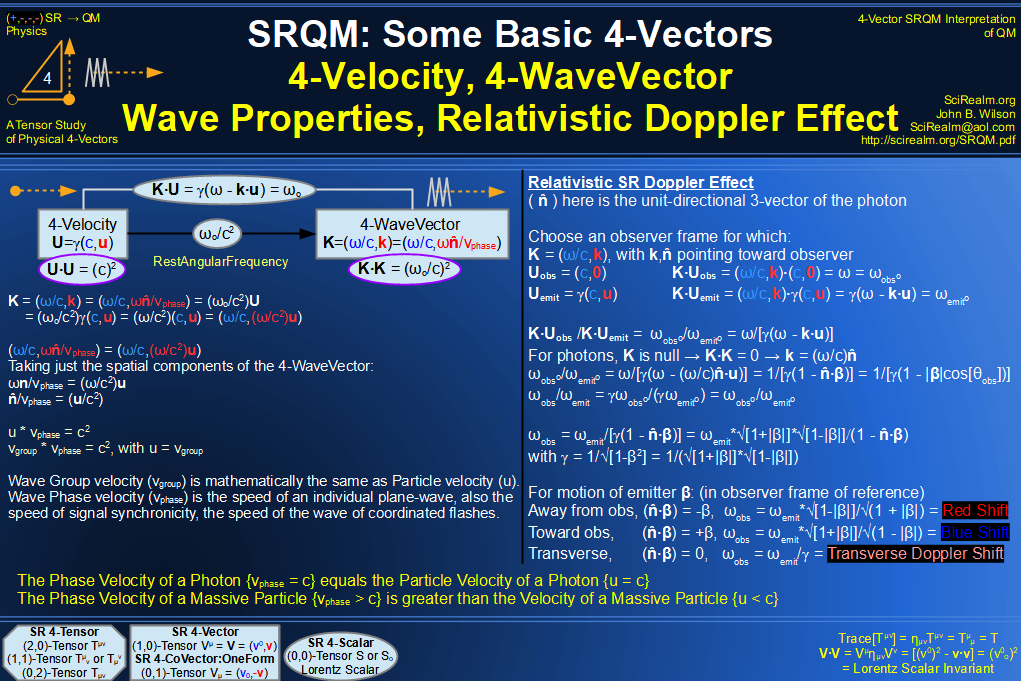SRQM 4-Vector : Four-Vector Wave-Particle Diagram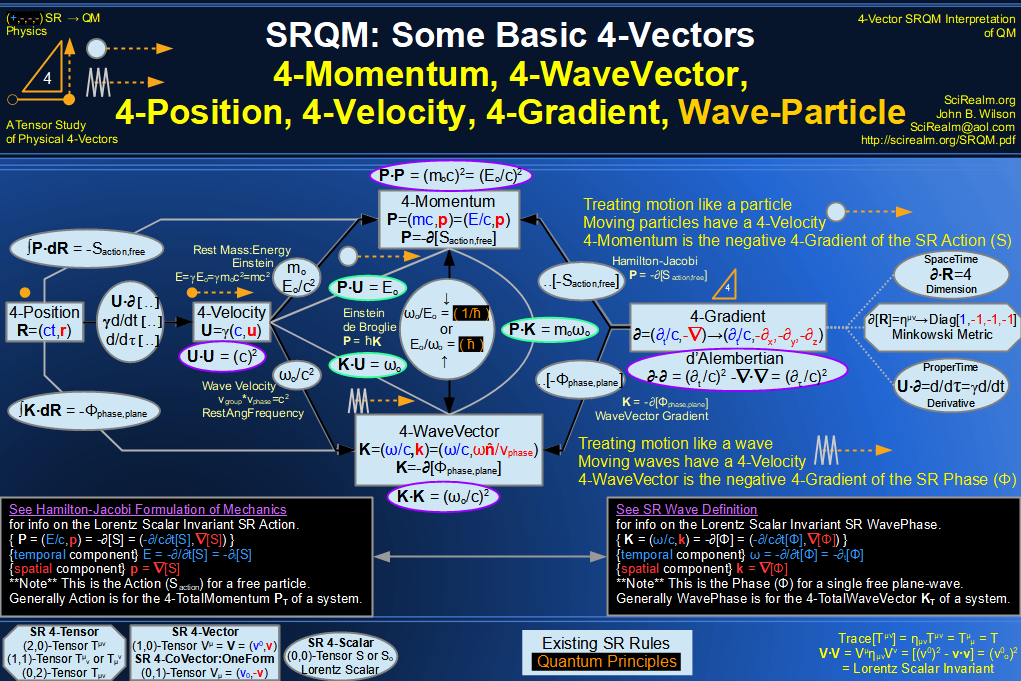SRQM 4-Vector : Four-Vector Compton Effect Diagram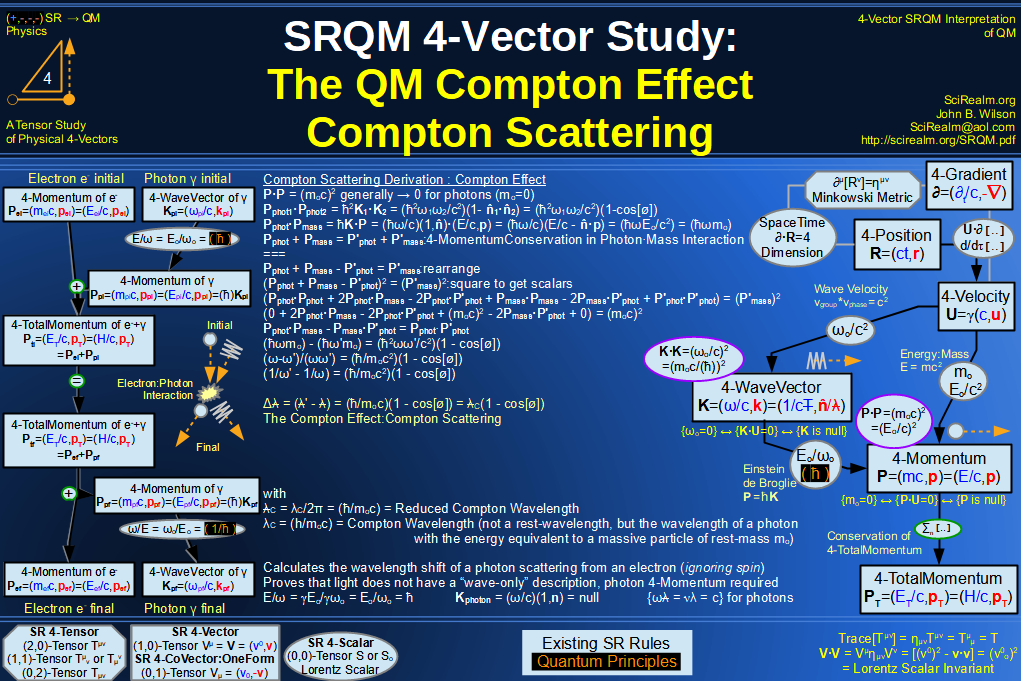SRQM 4-Vector : Four-Vector Aharonov-Bohm Effect Diagram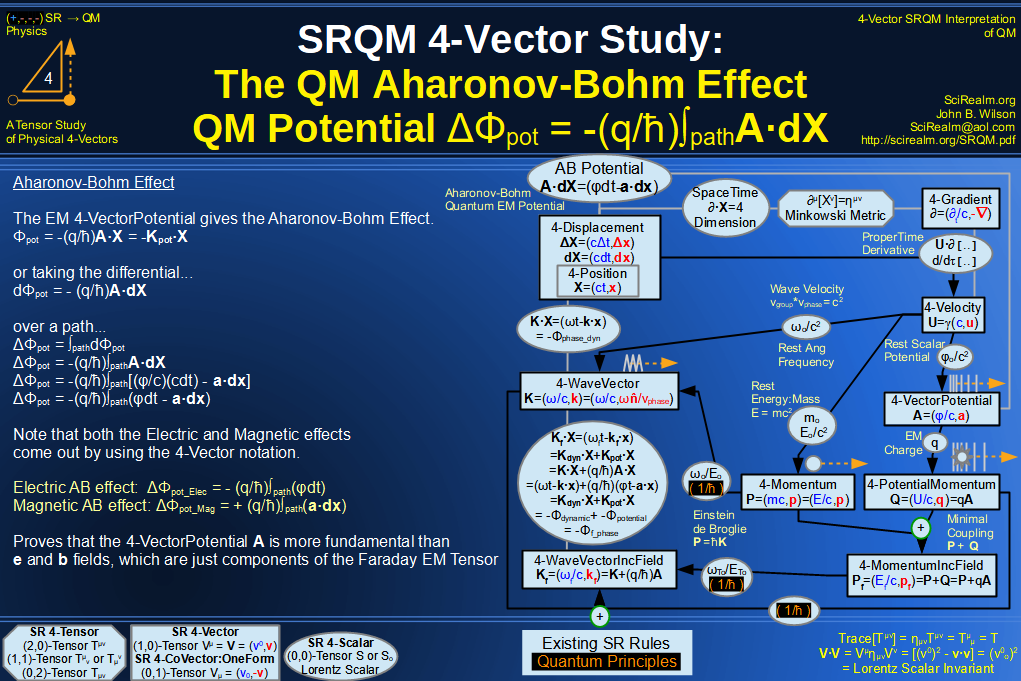SRQM 4-Vector : Four-Vector Josephson Junction Effect Diagram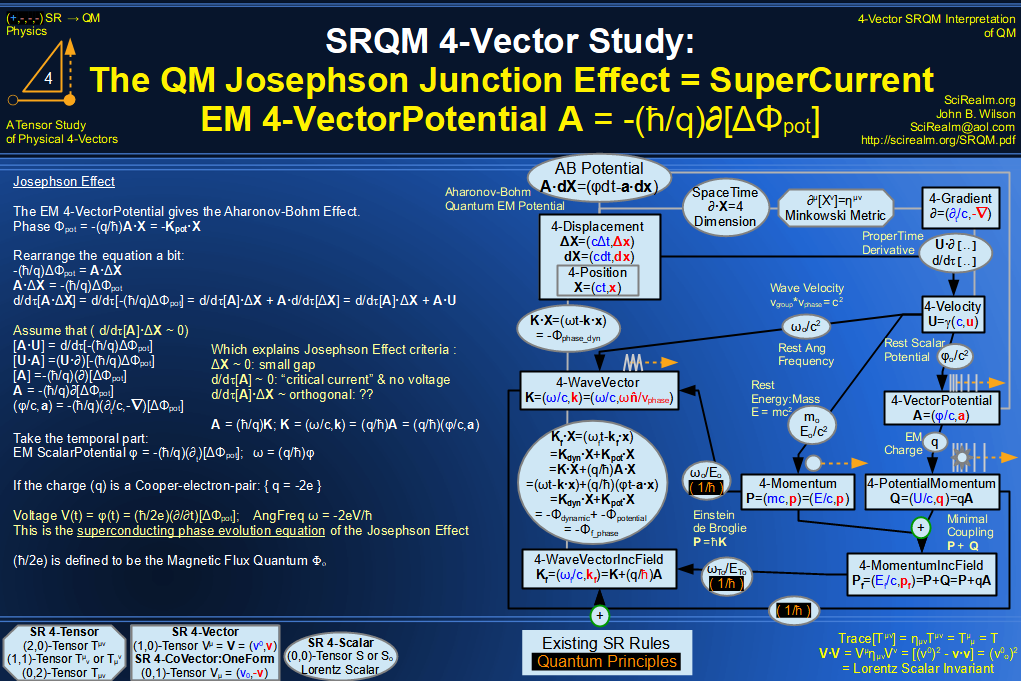SRQM 4-Vector : Four-Vector Hamilton-Jacobi vs Action, Josephson vs Aharonov-Bohm Diagram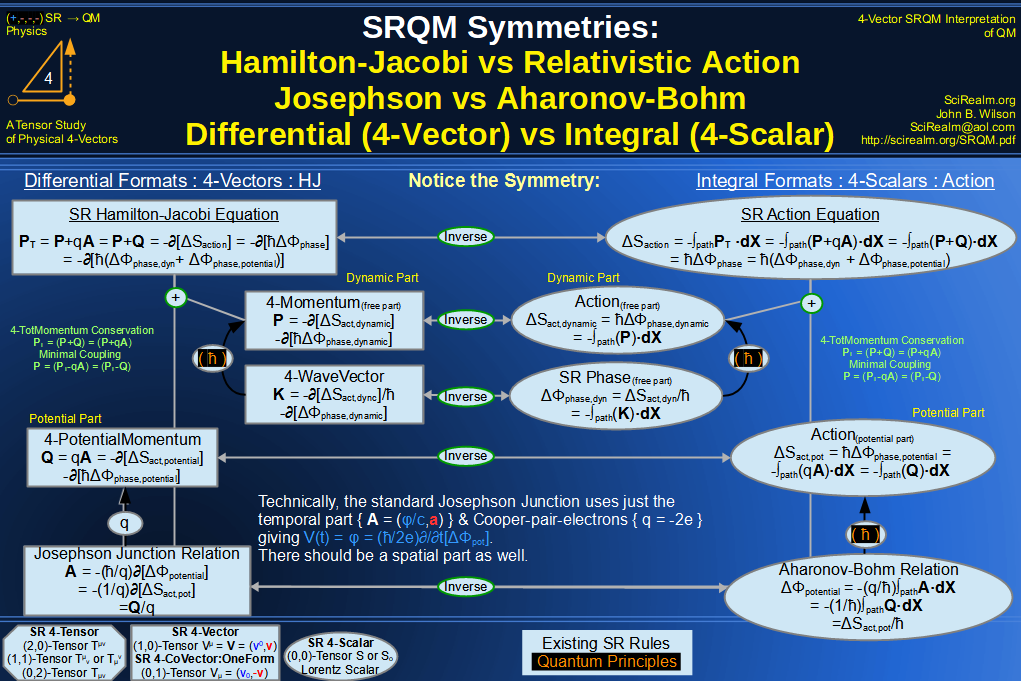SRQM 4-Vector : Four-Vector Motion of Lorentz Scalar Invariants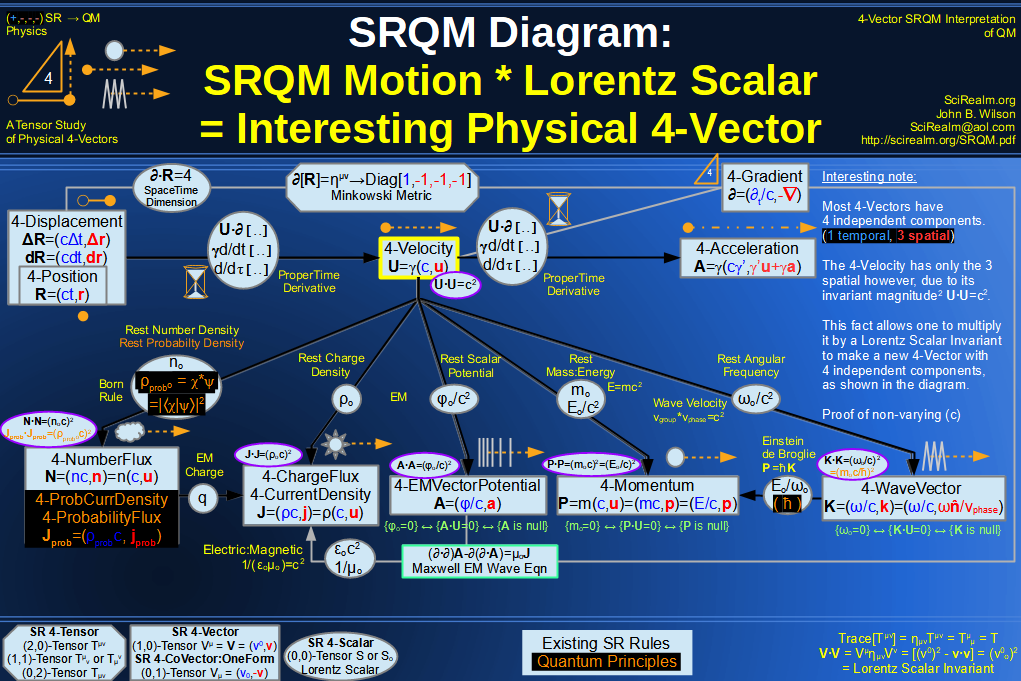SRQM 4-Vector : Four-Vector Motion of Lorentz Scalar Invariants, Conservation Laws & Continuity Equations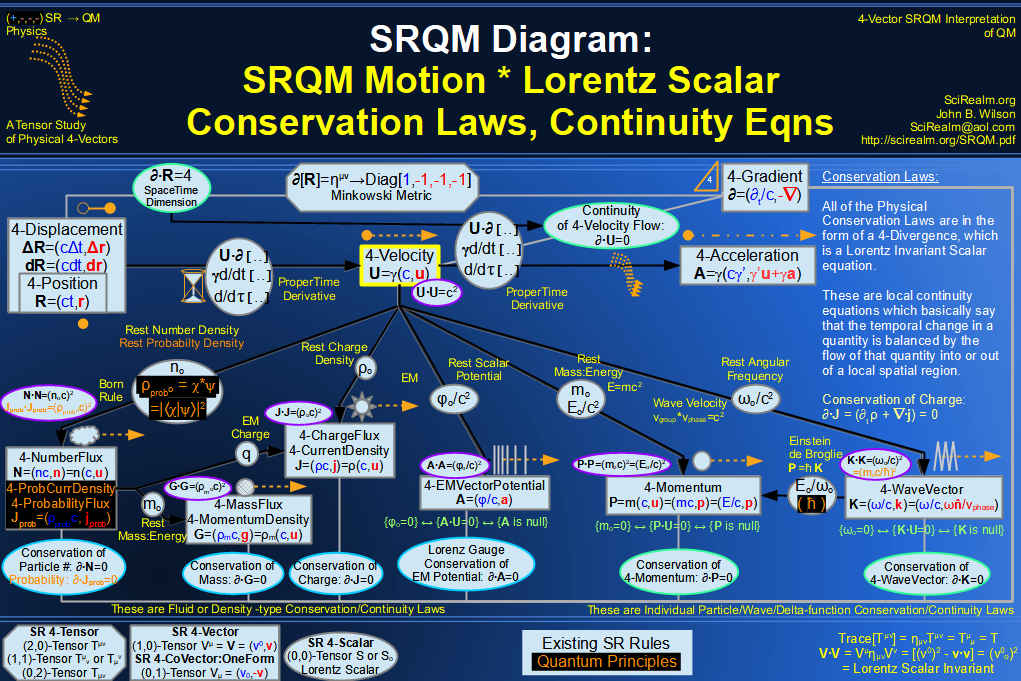SRQM 4-Vector : Four-Vector Speed of Light (c)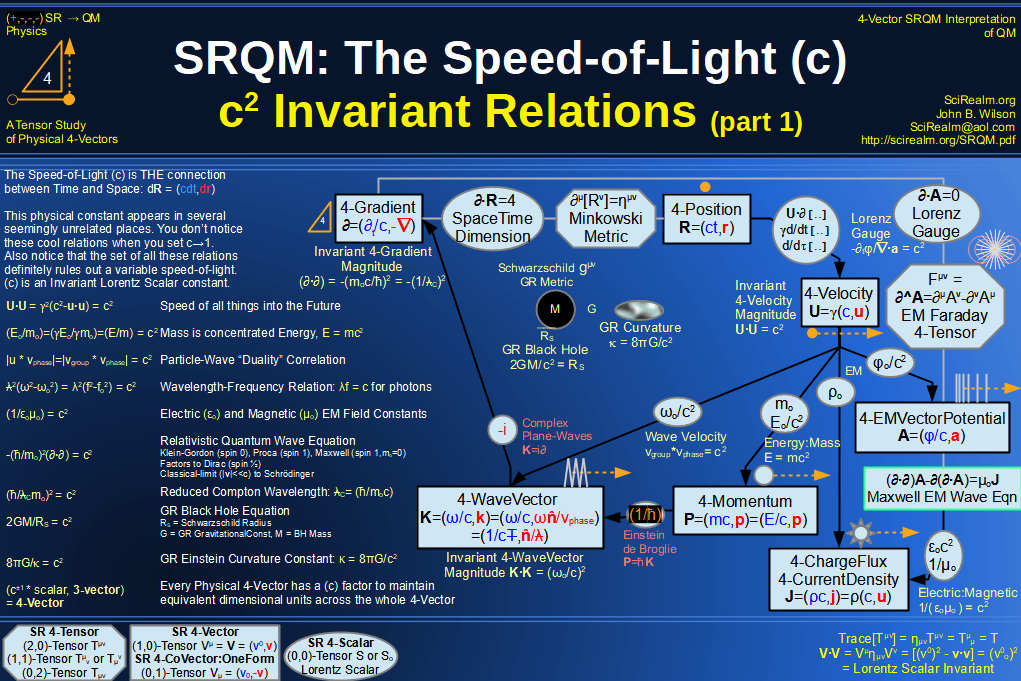SRQM 4-Vector : Four-Vector Minimal Coupling Conservation of 4-TotalMomentum)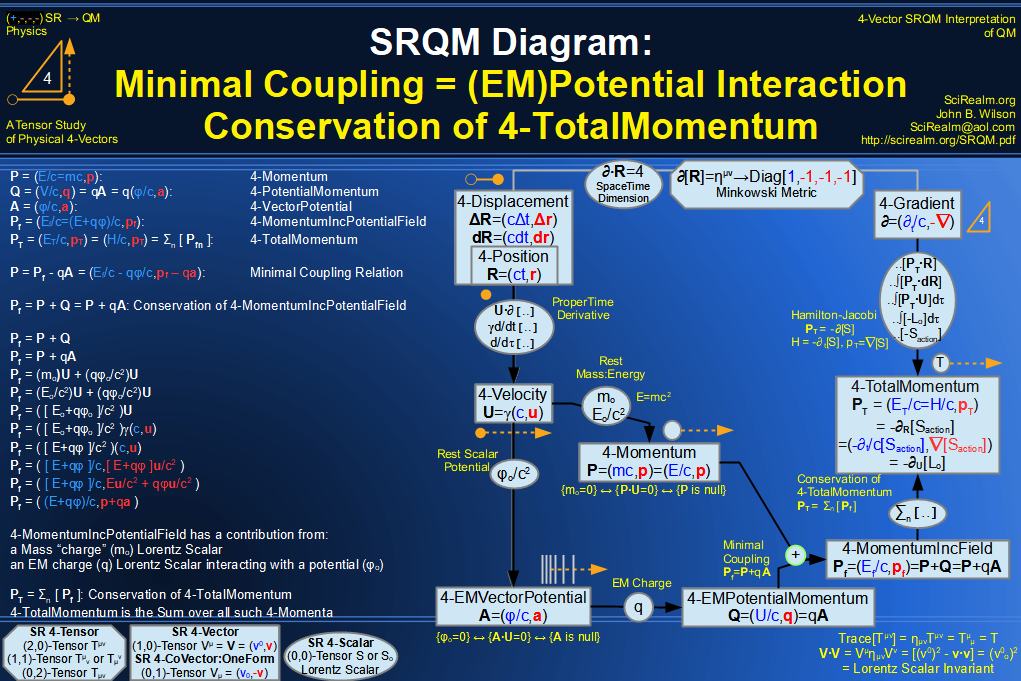SRQM 4-Vector : Four-Vector Relativistic Action (S) Diagram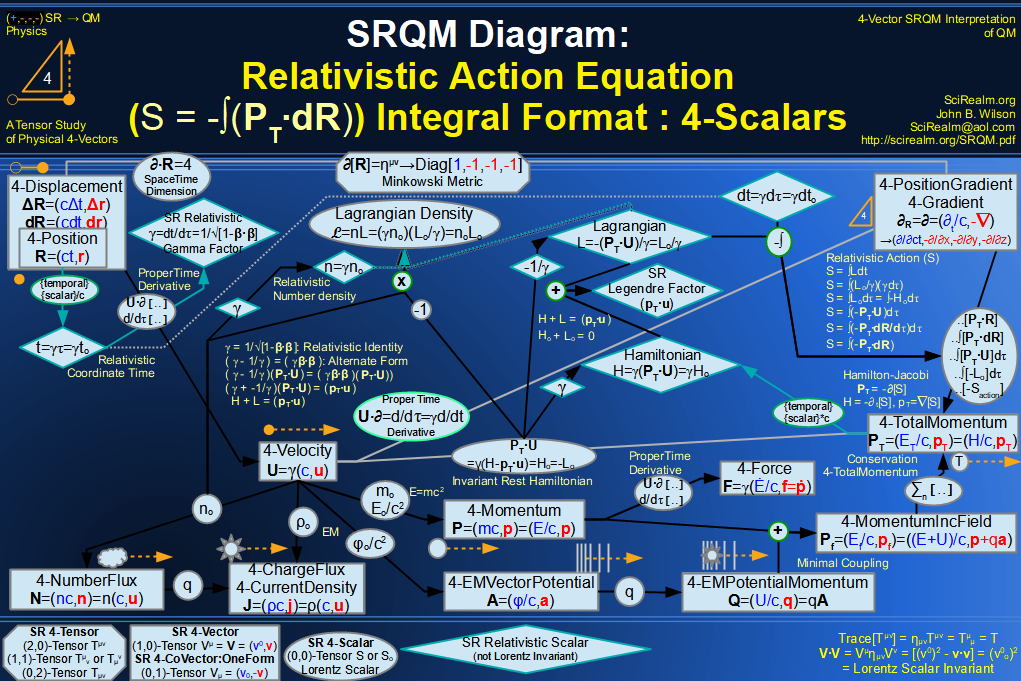SRQM 4-Vector : Four-Vector Relativistic Lagrangian Hamiltonian Diagram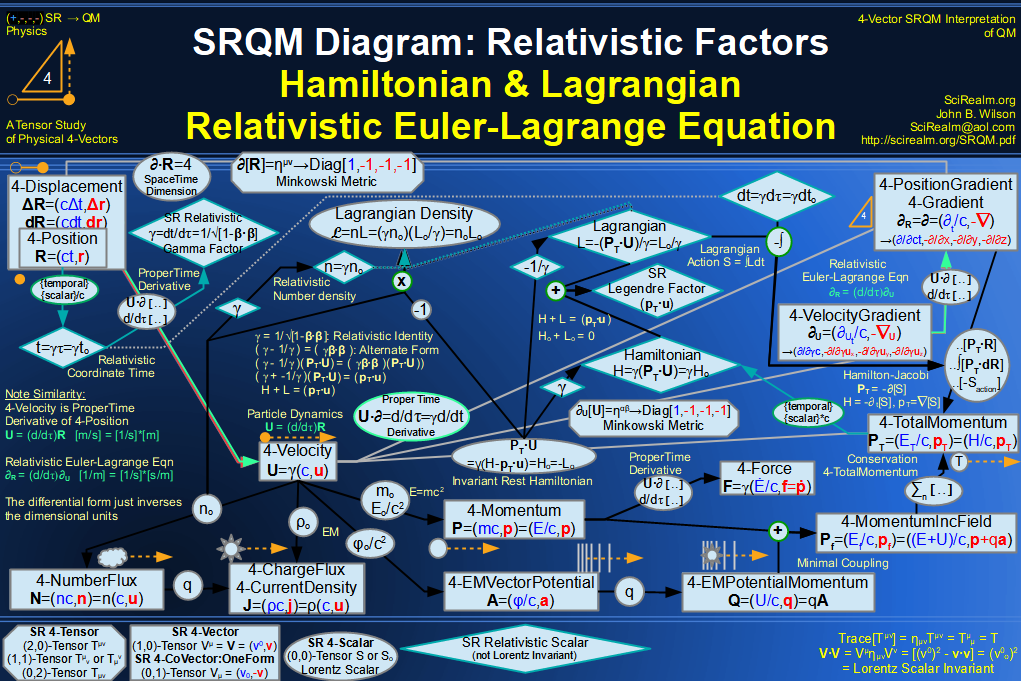SRQM 4-Vector : Four-Vector Relativistic Euler-Lagrange Equation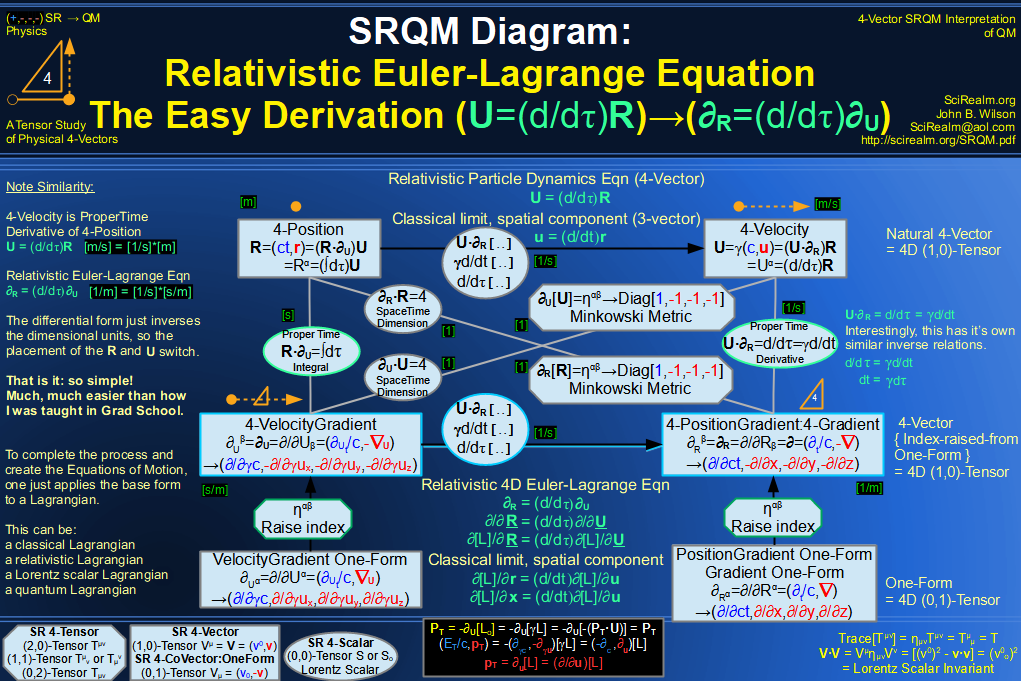SRQM 4-Vector : Four-Vector Relativistic EM Equations of Motion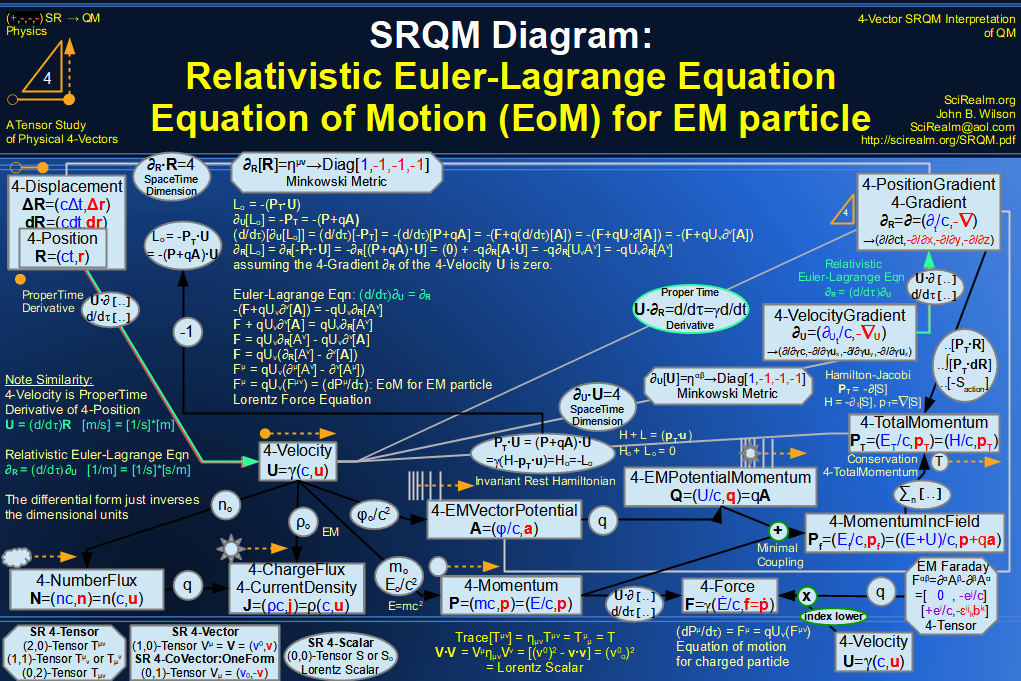SRQM 4-Vector : Four-Vector Einstein-de Broglie Relation hbar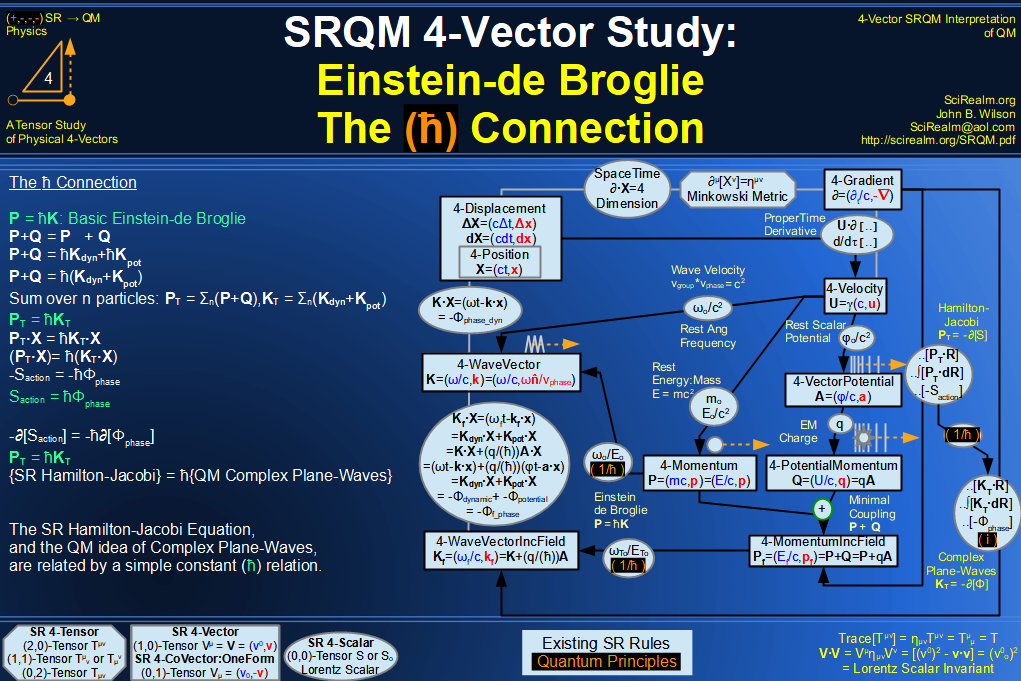SRQM 4-Vector : Four-Vector Quantum Canonical Commutation Relation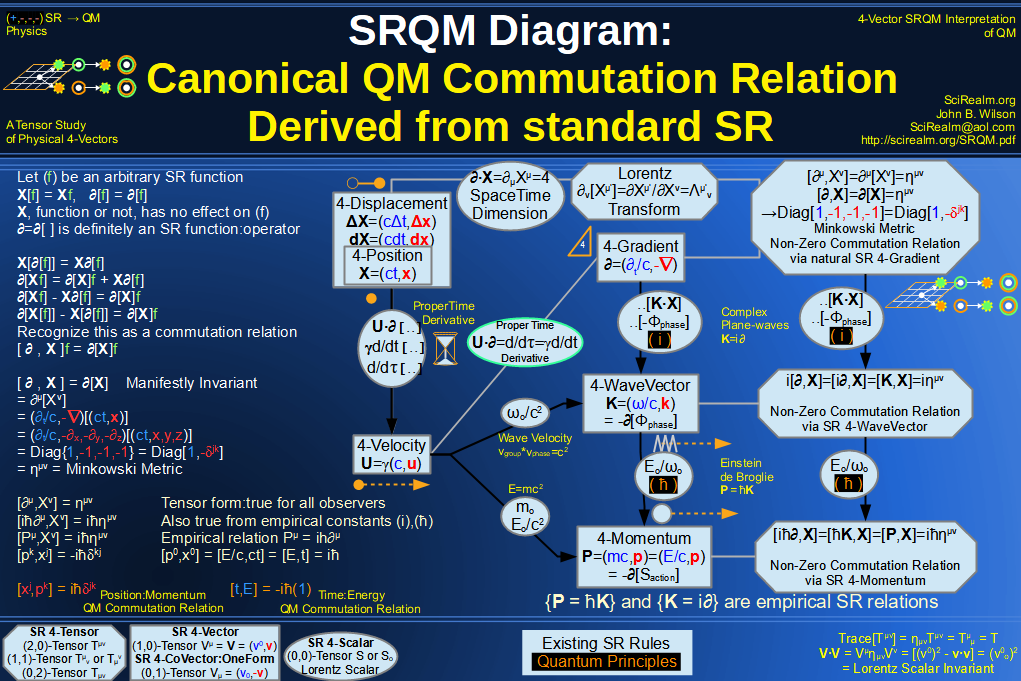SRQM 4-Vector : Four-Vector QM Schroedinger Relation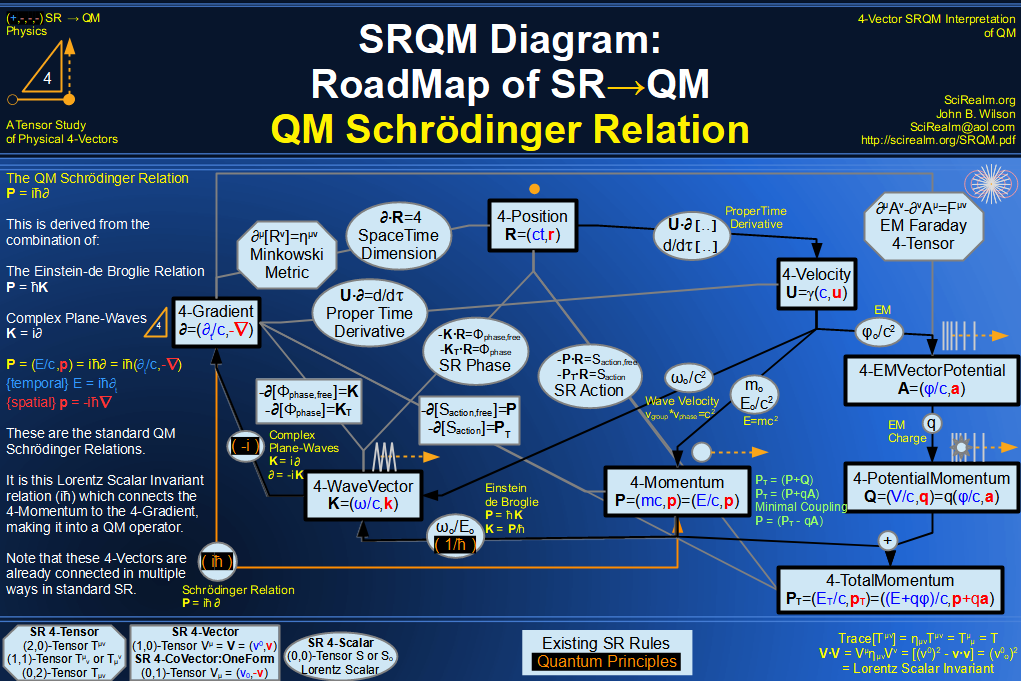SRQM 4-Vector : Four-Vector Quantum Probability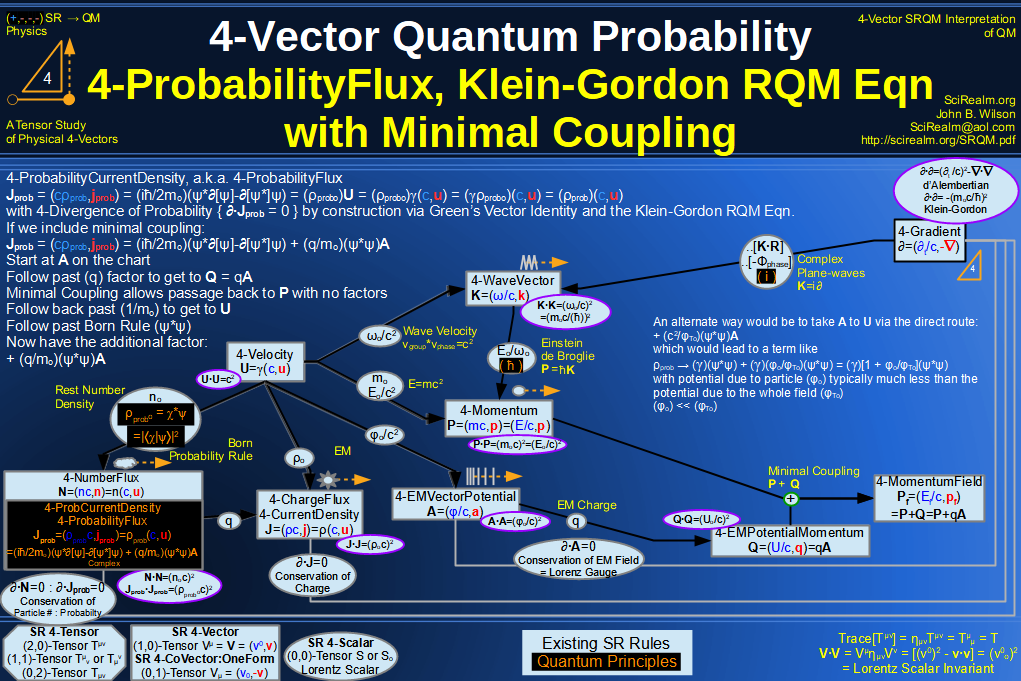SRQM 4-Vector : Four-Vector CPT Theorem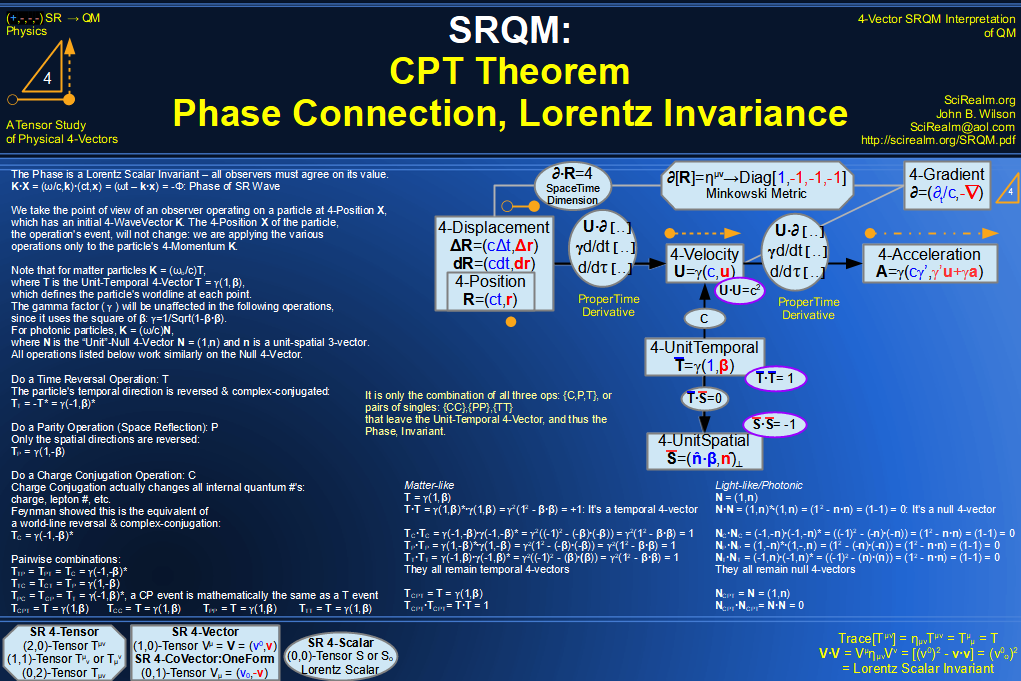SRQM 4-Vector : Four-Vector Lorentz Transforms Connection Map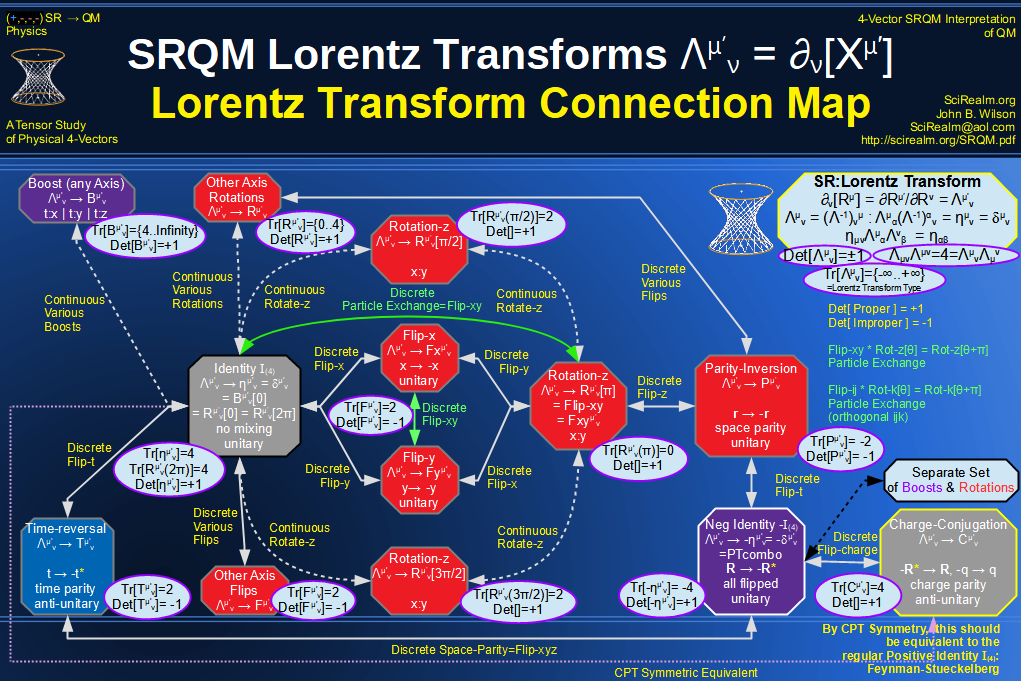SRQM 4-Vector : Four-Vector Lorentz Discrete Transforms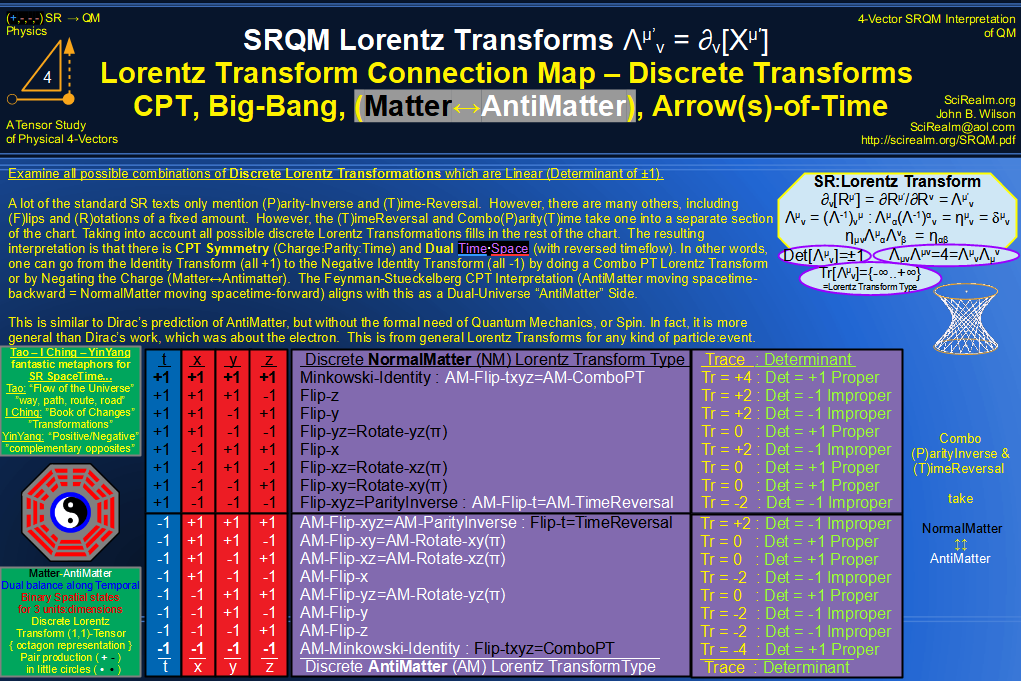SRQM 4-Vector : Four-Vector Lorentz Transforms - Trace Identification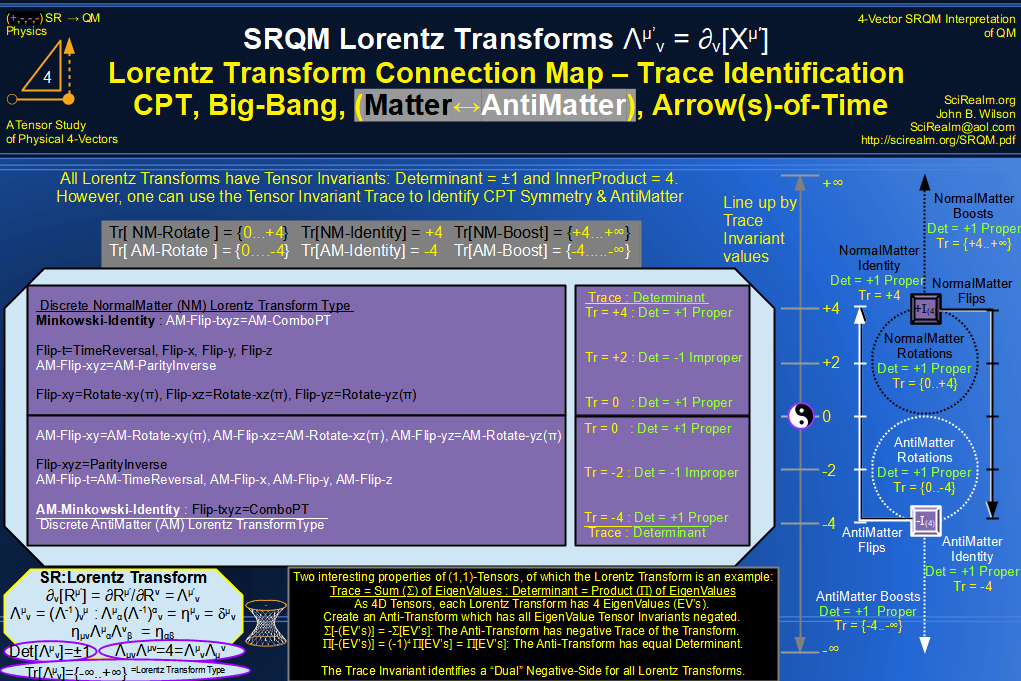SRQM 4-Vector : Four-Vector Lorentz Lorentz Transforms-Interpretations
CPT Symmetry, Baryon Asymmetry Problem Solution, Matter-Antimatter Symmetry Solution, Arrow-of-Time Problem Solution, Big-Bang!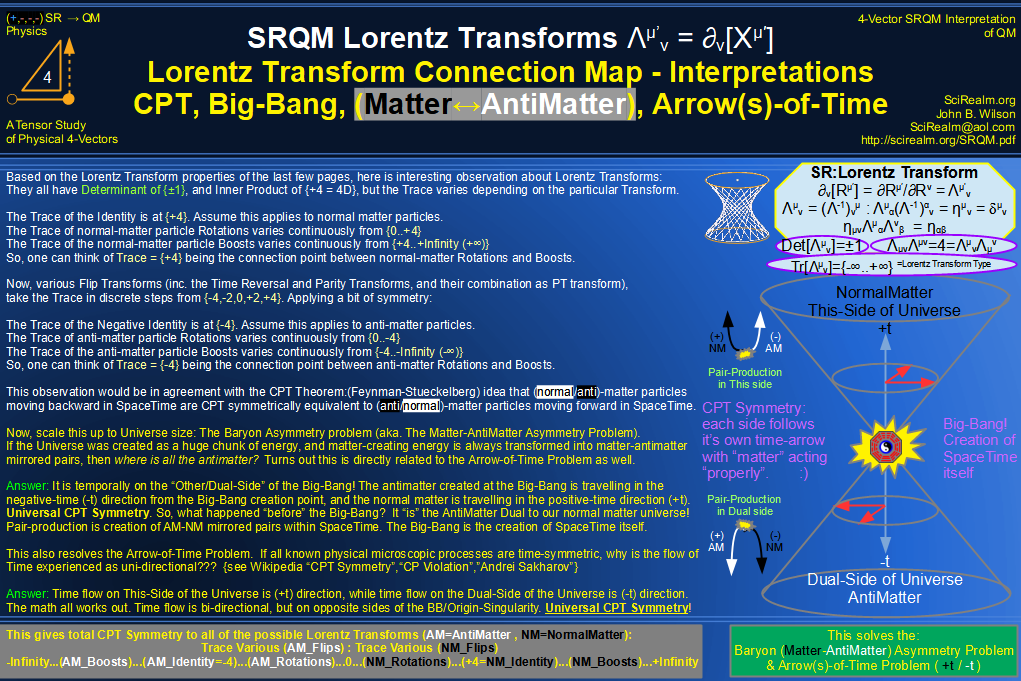See SRQM: QM from SR - The 4-Vector RoadMap (.html)
See SRQM: QM from SR - The 4-Vector RoadMap (.pdf)The Science Realm: John's Virtual Sci-Tech Universe John's Science & Math Stuff: | About John | Send email to John 4-Vectors | Ambigrams | Antipodes | Covert Ops Fragments | Cyrillic Projector | Forced Induction (Sums Of Powers Of Integers) | Fractals | Frontiers | JavaScript Graphics | Kid Science | Kryptos | Philosophy | Photography | Prime Sieve | QM from SR | QM from SR-Simple RoadMap | SR 4-Vector & Tensor Calculator | Quantum Phase | Quotes | RuneQuest Cipher Challenge | Scientific Calculator (complex capable) | Secret Codes & Ciphers | Science+Math | Sci-Pan Pantheist Poems | Stereograms | SuperMagicSqr4x4 | Turkish Grammar | Quantum Mechanics is derivable from Special Relativity See SRQM - QM from SR - Simple RoadMap (.html) See SRQM - QM from SR - Simple RoadMap (.pdf)